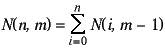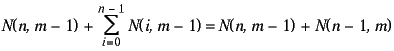A Dice Theorem
by Peter Meyer

How many ways are there of throwing two indistinguishable dice? The answer is 21. Why?

Answer: There are 6 ways of throwing the first die, and 6 ways of throwing the second die, and since the die throws are independent, there are 6*6 = 36 possibilities. But a 2 and a 3 is the same as a 3 and a 2 (if the dice are indistinguishable), so there are in fact less than 36 ways of throwing two dice. Of the 36 possibilities (when the dice are distinguishable) 6 are pairs of one number (1 plus 1, etc.). That leaves 30 where the dice have different numbers, so there are 30/2 = 15 possible results (when the numbers are different) if we do not distinguish one die from the other. These 15, plus the 6 results when the numbers are the same on each die, give 21.

Suppose instead of normal cubic dice we use octagonal dice, where each die has 8 faces (numbered 1 through 8). How many ways are there of throwing two indistinguishable octagonal dice? By the same reasoning as above we obtain 8 + (8*8 - 8)/2 = 36.

Suppose we use three cubic dice. This is more complicated because instead of equivalent pairs, e.g., (1,2) = (2,1), we now have equivalent triples, e.g. (1,2,3) = (1,3,2) = (2,1,3) = (2,3,1) = (3,1,2) = (3,2,1), which all count as one way of throwing three normal dice.

So let's now really complicate things and ask: How many ways are there of throwing n dice each with m faces? A difficult question.

Here is the answer:

The number of ways of throwing n dice each with m faces is

( n + m - 1)! / ( n! * ( m - 1 )! )

where x! is x factorial, i.e., x*(x-1)*(x-2)*...*3*2*1.

So the number of ways of throwing three cubic dice (n = 3, m = 6) is 8!/(3!*5!) = 8*7*6/6 = 56.

Are there more ways of throwing six octagonal dice (n = 6, m = 8) than there are ways of throwing eight cubic dice (n = 8, m = 6)?

Yes. One can throw six octagonal dice in 1,716 ways, but eight cubic dice in only 1,287 ways (1716/1287 = 4/3).

Here is the proof of the answer:

Theorem: Let N(n,m) denote the number of ways of throwing n dice each with m faces, then

N(n,m) = ( n + m - 1)! / ( n! * ( m - 1 )! )

The proof is in three parts.

 Lemma 1:Proof: The number of ways of throwing n dice with m faces is the sum of the number of ways of throwing n m's plus the number of ways of throwing n-1 m's and 1 non-m plus the number of ways of throwing n-2 m's and 2 non-m's ... plus the number of ways of throwing 0 m's and n nom-m's. Clearly this is equal to the sum of the number of ways of throwing 0 dice with m-1 faces plus the number of ways of throwing 1 die with m-1 faces plus the number of ways of throwing 2 dice with m-1 faces ... plus the number of ways of throwing n dice with m-1 faces. This is N(0,m-1) + N(1,m-1) + N(2,m-1) + ... + N(n,m-1), which proves the lemma.

 Lemma 2:Proof: By Lemma 1 we have that=We now state the theorem in the following form and prove it by induction on k:

Theorem: For all k ≥ 2, if n ≥ 1 and m ≥ 1 are such that n + m = k then
N(n,m) = ( n + m - 1)! / ( n! * ( m - 1 )! )

Proof: Consider k = 2. Suppose n + m = k, then n = 1 and m = 1. N(1,1) = 1 and
( 1 + 1 - 1)! / ( 1! * ( 1 - 1 )! ) = 1. Thus the theorem is true for k = 2.

Suppose the theorem is true for k - 1, then we shall show it is true for k.

Suppose n and m are such that n + m = k. By Lemma 2, N(n,m) = N(n,m-1) + N(n-1,m).
Since n + m - 1 = k - 1 and n - 1 + m = k - 1 we have that
N(n,m-1) = ( n + m - 2 )! / ( n! * ( m - 2 )! ) and
N(n-1,m) = ( n - 1 + m - 1 )! / ( ( n - 1 )! * ( m - 1 )! ).
Thus N(n,m-1) + N(n-1,m)
= (( n + m - 2)! * ( m - 1 )) / ( n! * ( m - 1)! )
+ ( n + m - 2 )! * n ) / ( n! * ( m - 1 )! )
= ( n + m - 2)! * ( n + m -1 ) / ( n! * ( m - 1 )! )
= ( n + m - 1)! / ( n! * ( m - 1)! )
which shows the theorem to be true for k. This completes the proof.

A note on dice:

Open Directory Project editor Ashay Dharwadker raised the question of whether there are only five possible values for m, namely, 4, 6, 8, 12 and 20, these being the number of faces on the five so-called Platonic solids (a.k.a. convex regular polyhedra), respectively the tetradhedron, the cube, the octagon, the dodecahedron and the icosahedron.

This is true provided we impose three conditions on the shape of a "die": (i) It is a 3-dimensional object, (ii) it is a convex polyhedron and (iii) the probability of a face turning up in a throw is the same for all faces.

In Euclidean spaces with dimension greater than 3 there are regular convex "polytopes", such as the hypercube, which consists of eight cubes, and the hypertetrahedron, which consists of five tetrahedra. But since we are not 4-dimensional beings we cannot throw 4-dimensional dice.

The second condition seems necessary to the concept of a die because although one can throw non-convex polyhedra (such as the four regular ones) it is not clear which of the faces can be said to be the face which results from a throw.

If we drop the requirement of regularity we find there are semiregular convex polyhedra, the so-called Archimedean solids, which we might use as dice. There are 13 of these. There is one which most people are familiar with, the so-called truncated icosahedron, which we encounter as soccer balls. This is formed from 12 regular pentagons and 20 regular hexagons, and so has 32 faces.

For use as a die an Archimedean solid must be such that opposite each face is a face, not a vertex, so that when it is thrown one face only will be uppermost. (This rules out the use of tetrahedra as dice.) The cuboctahedron, which has six square and eight triangular faces, has this property. In this case the number of faces is 14, different from the number of faces of any of the Platonic solids.

An interesting problem is: When a cuboctahedral die is thrown what is the probability of a square face resulting?

If the probability of a face of a certain type resulting depends on the relative area of that face (square vs. triangle) then one might reason as follows: Assuming a unit edge for the cuboctahedron the area of a square face is 1 and that of a triangular face is sqrt(3)/4 = 0.433, so the probability of a square face turning up is 6 / ( 6*1 + 8*sqrt(3)/4 ) = 0.634.  But it might be that the probability depends on the physical properties of cuboctahedral dice in motion and how exactly a die comes to rest on one face, in which case the question cannot be answered so simply.